# Ignoring Blank or Zero Cells with Conditional formatting

This post will guide you how to make conditional formatting ignore blank cells or zero cell in Excel. How do I force blank cells or zero cells to be ignored in conditional formatting in Excel. How to ignore blank cells or blank cells when applying conditional formatting in Excel.

## Ignoring Blank with Conditional Formatting

When you create conditional formatting rules for a list of selected data, and you want to ignore all Blank cells, How to achieve it. Just do the following steps:

#1 select the source data that you want to apply the conditional Formatting.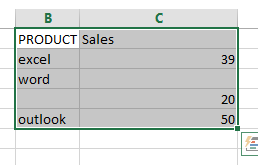#2 go to HOME tab, click Conditional Formatting command under Styles group. And select New Rule from the popup menu list. And the New Formatting Rule dialog will open.

#3 select Use a formula to determine which cells to format in the Select a Rule Type section.

#4 type the following formula into the Format values where this formula is true text box. Click Ok button.

=ISBLANK(B1)=TRUE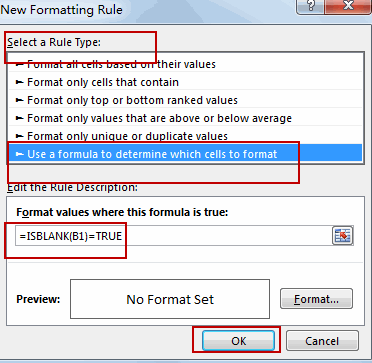Note: the B1 is the first cell of the selected range of cells.

## Ignoring zero with Conditional Formatting

If you want to ignore zero values with conditional Formatting, you can do the following steps:

#1 select the range of cells C2:C5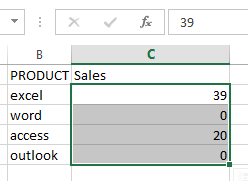#2 go to HOME tab, click Conditional Formatting command under Styles group, and select New Rule, and the New Formatting Rule dialog will open.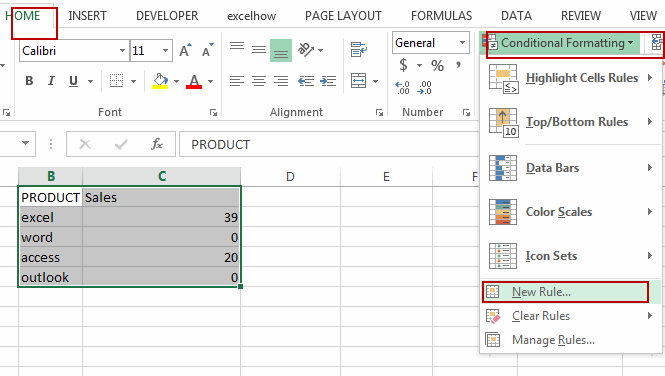#3 select Use a formula to determine which cells to format in the Select a Rule Type section.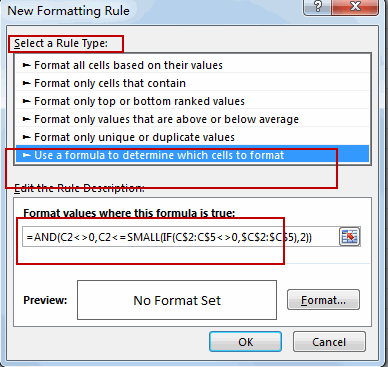#4 type the following formula into the Format values where this formula is true text box.

=AND(C2<>0,C2<=SMALL(IF(C\$2:C\$5<>0,\$C\$2:\$C\$5),2))

#5 click Format button, and switch to Fill tab in Format Cells dialog, select one color as the background color. Click Ok button.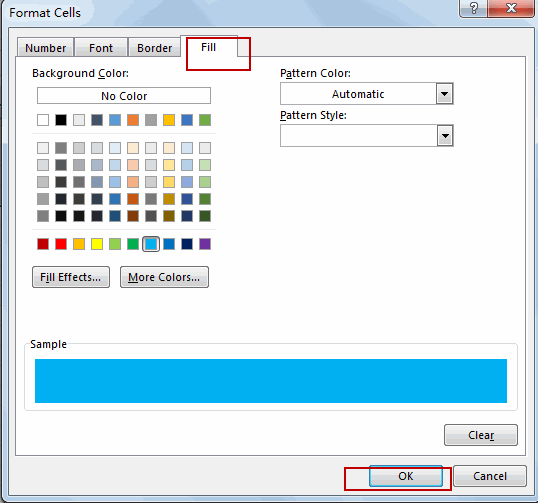#6 click Ok button. You will see that all zero values are ignored by conditional formatting.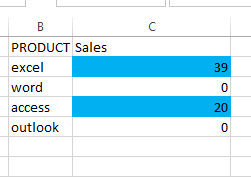### Related Functions

• Excel ISBLANK function
The Excel ISBLANK function returns TRUE if the value is blank or null.The syntax of the ISBLANK function is as below:= ISBLANK (value)…
• Excel IF function
The Excel IF function perform a logical test to return one value if the condition is TRUE and return another value if the condition is FALSE. The IF function is a build-in function in Microsoft Excel and it is categorized as a Logical Function.The syntax of the IF function is as below:= IF (condition, [true_value], [false_value])….
• Excel SMALL function
• The Excel SMALL function returns the smallest numeric value from the numbers that you provided. Or returns the smallest value in the array.The syntax of the SMALL function is as below:=SMALL(array,nth) …
• Excel AND function
• The Excel AND function returns TRUE if all of arguments are TRUE, and it returns FALSE if any of arguments are FALSE.The syntax of the AND function is as below:= AND (condition1,[condition2],…)…